# Fill in the blanks.

Question:

Fill in the blanks.

(i) If 3 persons can do a piece of work in 4 days, then 4 persons can do it in ......... days.

(ii) If 5 pipes can fill  tank in 144 minutes, then 6 pipes can fill it in ......... minutes.

(iii) A car covers a certain distance in 1 hr 30 minutes at 60 km per hour. If it moves at 45 km per hour, it will take ......... hours.

(iv) If 8 oranges cost Rs 20.80, the cost of 5 oranges is Rs .........

(v) The weight of 12 sheets of a paper is 50 grams. How many sheets will weigh 500 grams?

Solution:

(i) Let x be the number of days taken by 4 persons to complete the work.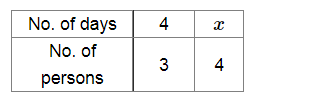Clearly, more workers will take less number of days.

So, it is a case of inverse proportion.

Now, $4 \times 3=x \times 4$

$\Rightarrow x=3$

Therefore, 4 persons can do the piece of work in 3 days.

(ii) Let x min be the time taken by 6 pipes to fill the tank.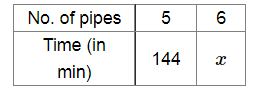Clearly, more number of pipes will take less time to fill the tank.

So, it is a case of inverse proportion.

Now, $5 \times 144=6 \times x$

$\Rightarrow x=\frac{5 \times 144}{6}$

$\Rightarrow x=120 \mathrm{~min}$

∴ 6 pipes can fill the tank in 120 min.

(iii) Let x min be the time taken by the car travelling at 45 km/h.

Now, 1 h 30 min = (60+30) min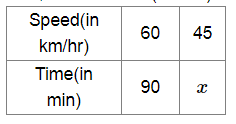Clearly, a car travelling at a less speed will take more time.

So, it is a case of inverse proportion.

Now, $60 \times 90=45 \times x$

$\Rightarrow x=\frac{60 \times 90}{45}$

$\Rightarrow x=120 \mathrm{~min}=2 \mathrm{~h}$

∴ The car will take 2 h if it travels at a speed of 45 km/h.

(iv) Let Rs be the cost of 5 oranges.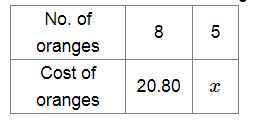Clearly, less number of oranges will cost less.

So, it is a case of direct variation.

Now, $\frac{8}{20.80}=\frac{5}{x}$

$\Rightarrow x=\frac{5 \times 20.80}{8}$

$\Rightarrow x=13$

∴ The cost of 5 oranges is Rs 13.

(v) Let x be the number of sheets that weigh 500 g.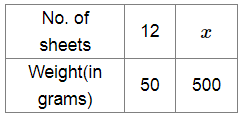More number of sheets will weigh more.

So, it is a case of direct variation.

Now, $\frac{12}{50}=\frac{x}{500}$

$\Rightarrow x=\frac{12 \times 500}{50}$

$\Rightarrow x=120$

∴ 120 sheets will weigh 500 g.